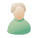# سرعة الجسم تحت تأثير قوة فيزياء الصف الاول الثانوى المطور

28072013##سرعة الجسم تحت تأثير قوة فيزياء الصف الاول الثانوى المطور

سرعة الجسم تحت تأثير قوة فيزياء الصف الاول الثانوى المطور
Note: Put your calculator into radians mode before using circular motion equations!
Remember Newton's First law?
"If an object continues in a straight line at constant velocity, all forces acting on the object are balanced."
Or another way of putting it...
"An object at rest tends to stay at rest and an object in motion tends to stay in motion with the same speed and in the same direction unless acted upon by an unbalanced force."
Objects moving in circular motion clearly aren't going in a straight line so the forces can't be balanced.
There is a resultant force. This is called the centripetal force.
The centripetal force is always directed towards the centre of the circle (along the radius of the circle).
(There is no such thing as centrifugal force, so don't mention it in your exams!)
Angular acceleration and centripetal force
If an object is moving with constant speed in circular motion, it is not going at constant velocity. That's because velocity is a vector. Although its magnitude remains the same, its direction varies continuously.
Because its velocity is changing, we say it is accelerating. That sounds odd! You've got something that's accelerating but not speeding up (or slowing down)! But it is experiencing an unbalanced (or resultant) force. Thats what makes it change direction.
This resultant force, the centripetal force, causes the centripetal acceleration. It is always at 90° to the direction of movement of the object - and that's why the object doesn't speed up!
If you think about a satellite orbiting the Earth, the force of gravity provides the centripetal force and acceleration. The satellite is basically falling towards the earth but does so at the same rate as the Earth curves away from it!
Centripetal acceleration can be calculated using:Where:
a = centripetal acceleration (m/s2)
v = velocity (m/s)
r = radius of the circle (m)
And from Newton's Second Law:
F = ma, soThis is an equation for centripetal force.
Example:
A car of mass 750kg moves around a circular track of radius 50m with a speed of 10ms-1. What's the centripetal force on the car and which direction does it act in?
Answer:The direction of all centripetal forces is always towards the centre of the circle.
Vertical circles
When an object is moving in circles that are vertical, the weight of the object has to be taken into consideration.
Example:
An object of mass 2kg is attached to the end of a string length 1m and whirled in a vertical circle at a constant speed of 4ms-1. Find the tension in the string at the top and the bottom of the circle. Assume gravity = 10 m/s2
Answer:
The centripetal force is the resultant of all the other forces acting on the object. The only forces acting are the tension in the string, T, and weight, W, due to gravity.At the top:
The tension and weight are both acting down. Hence,
Centripetal force = tension + weight
Fc = T + W
So,
T = Fc - W =At the bottom:
The tension acts up and the weight acts down. Hence,
Centripetal force = tension - weight
Fc = T - W
So,
T = Fc + W =جزاك الله خيراجزاكم الله خيرا

صلاحيات هذا المنتدى:
لاتستطيع الرد على المواضيع في هذا المنتدى